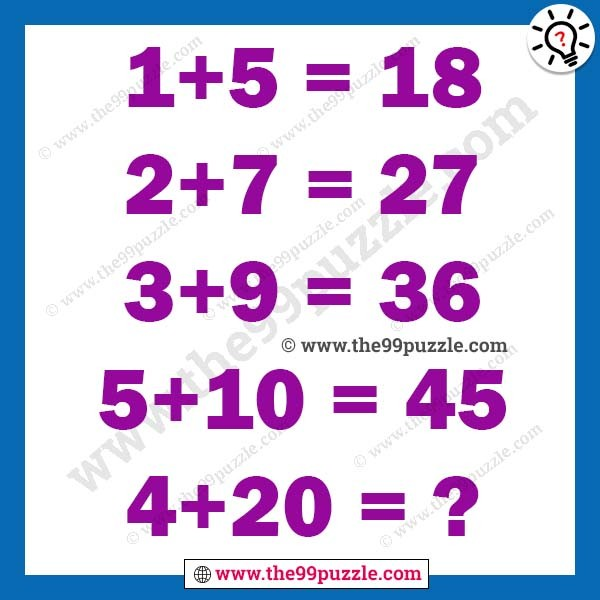# Math tricky riddle with answer – Puzz114

Can you solve the Math tricky riddle? This puzzle will challenge your brain. This math logic puzzle is for all school going students. Solving this math tricky riddle will help you to test your logical reasoning and mathematical skills. Can you find the value of this equation?

1+5=18

2+7=27

3+9=36

5+10=45

4+20=?1+5=6×3=18

2+7=9×3=27

3+9=12×3=36

5+10=15×3=45

4+20=24×3=72